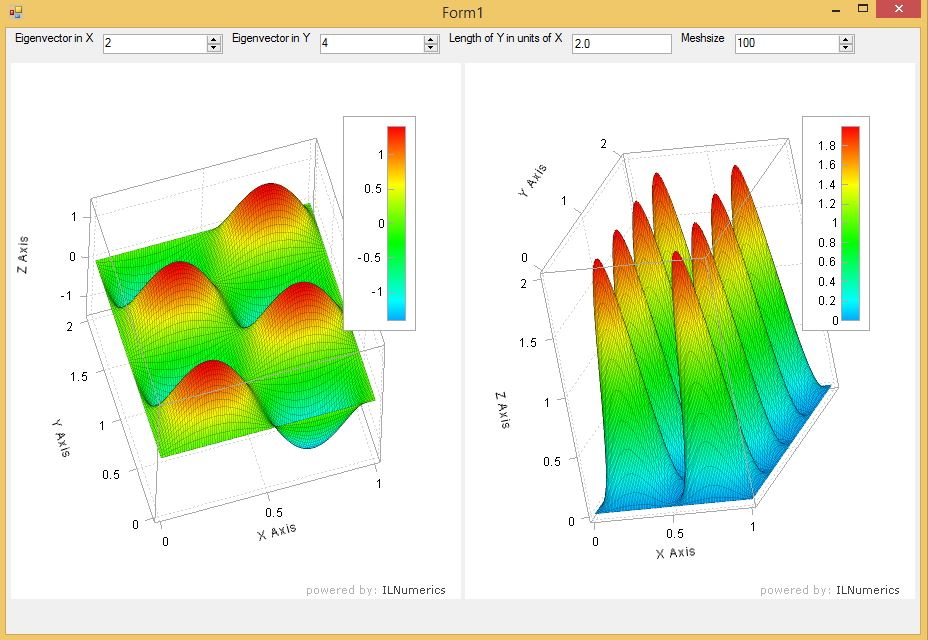# Tag Archives: Quantum Mechanics

## ILNumerics for Scientists – Going 3D

### Recap

Last time I started with one of the easiest problems in quantum mechanics: the particle in a box. This time I’ll add 1 dimension and we’ll see a particle in a 2D box. To visualize its wave function and density we need 3D surface plots.

### 2D Box

This time we have a particle that is confined in a 2D box. The potential within the box is zero and outside the box infinity. Again the solution is well-known and can be found on Wikipedia. This time the state of the wave function is determined by two numbers. These are typically called quantum numbers and refer to the X and the Y direction, respectively.

The absolute size of the box doesn’t really matter and we didn’t worry about it in the 1D case. However, the relative size of the length and the width make a difference. The solution to our problem reads

$\Psi_{n,k}(x,y) = \sqrt{\frac{4}{L_x L_y}} \cdot \sin(n \cdot \pi \cdot x / L_x) \cdot \sin(k \cdot \pi \cdot y / L_y)$

### The Math

Very similar to the 1D case I quickly coded the wave function and the density for further plotting. I had to make sure that the arrays are fit for 3D plotting, so the code looks a little bit different compared to last post’s

     public static ILArray<double> CalcWF(int EVXID, int EVYID, double LX, double LY, int MeshSize)
{
ILArray<double> X = linspace<double>(0, LX, MeshSize);
ILArray<double> Y = linspace<double>(0, LY, MeshSize);

ILArray<double> Y2d = 1;
ILArray<double> X2d = meshgrid(X, Y, Y2d);

ILArray<double> Z = sqrt(4.0 / LX / LY) * sin(EVXID * pi * X2d / LX) * sin(EVYID * pi * Y2d / LY);

return Z.Concat(X2d,2).Concat(Y2d,2);
}


Again, this took me like 10 minutes and I was done.

### The Visualization

This time the user can choose the quantum numbers for X and Y direction, the ratio between the length and the width of the box and also the number of mesh points along each axis for plotting. This makes the visualization panel a little bit more involved. Nevertheless, it’s still rather simple and easy to use. This time it took me only 45 minutes – I guess I learned a lot from last time.

### The result

Here is the result of my little program. You can click and play with it. If you’re interested, you can download the Particle2DBox source code. Have fun!This is a screenshot of the application. I chose the second quantum number along the x axis and the fourth quantum number along the y axis. The box is twice as long in y direction as it is in x direction. The mesh size is 100 in each direction. On the left hand side you see the wave function and on the right hand side the probability density.

## ILNumerics for Scientists – An easy start

### Motivation

I’ve been working as a scientist at universities for 10 years before deciding to go into industry. The one thing I hated most was coding. At the end of the day coding for scientists is like running for a football player. Obviously, you need it but it’s not what you’re here for.

I really dreaded the coding and the debugging. So much precious time for something that was so clear on paper and I just wanted the solution of my equations to see whether my idea made sense or not. More often than not scientists find that their idea was not so great and now they had spent so much time coding just to find out that the idea didn’t work. Continue reading ILNumerics for Scientists – An easy start